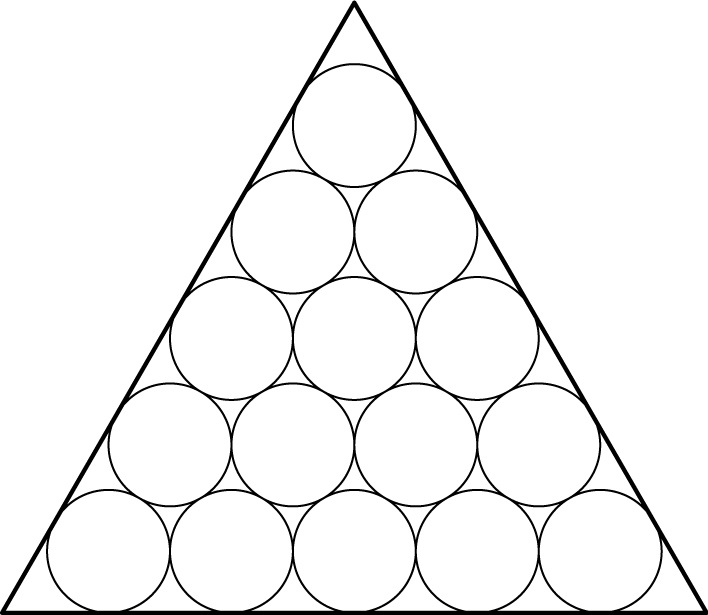# Circles Inscribed In a Triangle

Geometry Level 3Fifteen unit circles are inscribed in an equilateral triangle in such a way that each circle is externally tangent to its neighbors. What is the area of the triangle (in $\text{units}^2$)?

×